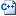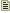# `phys_slideconstraint`class hierarchy
CPhysSlideConstraint defined in`physconstraint.cpp`
CPhysConstraint
CLogicalEntity
CServerOnlyEntity
CBaseEntity

`phys_slideconstraint` is a point entity available in all  games. It is a constraint that constrains an entity along a line segment.

## Keyvalues

Sliding Axis `(slideaxis)` <vector>
Axis of the constraint. Usually set with the Hammer helper tool.
Friction `(slidefriction)` <float>
Resistance/friction in the constraint
Load Scale `(SystemLoadScale)` <float>
Scale of the mass load connected to this constraint (1=just the objects directly connected)
Minimum Sound Velocity `(minSoundThreshold)` <float>
When travelling below this many units/sec, will not play any sound.
Full Sound Velocity `(maxSoundThreshold)` <float>
When travelling at this speed or above, will play sound at full volume.
Travel sound (forward) `(slidesoundfwd)` <sound>
Play this sound when travelling forward on helper axis
Travel sound (backward) `(slidesoundback)` <sound>
Play this sound when travelling backward on helper axis
Reversal sound threshold (small) `(reversalsoundthresholdSmall)` <float>
When accelerating by more than this many units/sec^2 opposite to direction of travel, play the small reversal sound.
Reversal sound threshold (medium) `(reversalsoundthresholdMedium)` <float>
When accelerating by more than this many units/sec^2 opposite to direction of travel, play the medium reversal sound.
Reversal sound threshold (large) `(reversalsoundthresholdLarge)` <float>
When accelerating by more than this many units/sec^2 opposite to direction of travel, play the large reversal sound.
Reversal sound (small) `(reversalsoundSmall)` <sound>
Play this sound when making a hard reverse over the small threshold but less than medium
Reversal sound (medium) `(reversalsoundMedium)` <sound>
Play this sound when making a hard reverse over the medium threshold but less than large
Reversal sound (large) `(reversalsoundLarge)` <sound>
Play this sound when making a hard reverse over the large threshold

TwoObjectPhysics:
Entity 1 `(attach1)` <targetname>
Entity 2 `(attach2)` <targetname>
The entities to constrain. Leave one or the other field blank to constrain to the world.Note:Only one entity will be constrained, even if several share the given targetname.
Constraint System Manager `(constraintsystem)` <targetname>
A `phys_constraintsystem` that this constraint should be a part of. This avoids the "jiggling" caused by constraints applied to the same set of entities fighting with each other.
Force Limit to Break `(forcelimit)` <float>
Impact force required to break the constraint, in pounds. 0 means infinite. A way of calculating this is to set it to the weight of an object that would break the constraint if it were resting on its objects.
Torque Limit to Break `(torquelimit)` <float>
Torque required to break the constraint, in pounds*inches. 0 means infinite. A way of calculating this is to multiply any reference mass by the resting distance (from the center of mass of the object) needed to break the constraint.
Play Sound on Break `(breaksound)` <string>
A sound played when the constraint is broken.
Follow teleport distance `(teleportfollowdistance)` <float>
If one constrained object teleports more than this many units away, the other will teleport with it.

Targetname:
Name `(targetname)` <string>
The targetname that other entities refer to this entity by.

## Flags

•  [`1`] : No Collision until break
•  [`2`] : Limit Endpoints

## Inputs

`SetVelocity` <float>
Set linear velocity along the constraint

TwoObjectPhysics:
`Break`
Force the constraint to break.
`TurnOn`
Enable the constraint; do this if the constrained objects don't exist when it spawns. Note that broken constraints cannot be turned back on as they have been deleted.
`TurnOff`
Disable the constraint.

## Outputs

TwoObjectPhysics:
`OnBreak`
Fired when the constraint breaks.